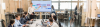Quantitative Foundations of Risk Management
Quantitative Foundations of Risk ManagementTraining Activity Rate
Trainer/s
اسامه شقير
Training activity Hours
12
Training activity Date
-
Training Activity Days
Monday
Tuesday
Wednesday
Thursday
Start and End Time
17:00 - 20:00
Training Activity Classification
Risk
Course Language
English
Methodology
In class
City
Amman
Type of Training
short courses
Price For Jordanian
96 JOD
Price For Non Jordanian
240 US\$Outcomes

- By the end of this training course, trainees will be able to :
-    Describe independent events and mutually exclusive events and explain the difference between independent events and conditionally independent events.
-    Calculate the probability of an event for a discrete probability function and calculate a conditional probability.
-    Define and distinguish between volatility, variance, correlation and covariance.
-    Distinguish between important distribution functions (e.g. Uniform, Normal, Lognormal Distribution, Student’s t, Binomial, Poisson)
-    Compute and interpret basic statistical measures relating to risk management (e.g. mean, standard deviation, kurtosis, correlation. etc.).
-    Construct simple and multiple liner regression and be able to apply these tools in finance.
-    Estimate simple volatility using historical financial data and identify the issues with traditional volatility estimation methods.

Target Group

-  Professionals working in:
-    Treasury.
-    Risk .
-    Capital Management.
-    Regulatory Compliance.
-    Audit.

General Goal

This module introduces the basic knowledge of probability and statistics, regression and time series analysis.  This knowledge serves as the foundation quantitative techniques that is useful in risk management. The broad knowledge points covered in this module include the following:

-       Probability Theory in Finance

-       Statistics

-       Linear regression with single and multiple variables

-       Volatility analysis and forecasting

Contents

-    Probability Theory in Finance:
-    Definition and rules:
-    Continuous and discrete data.
-    Types of discrete Distribution.
-    The Binomial Distribution.
-    The Poisson Distribution.
-    The Uniform Distribution.
-    Types of Continuous Distribution:
-    The Normal Distribution.
-    The Lognormal Distribution.
-    Probability Distributions:
-    Random Variables.
-    Probability Density Functions and Histograms.
-    Joint Distributions (Covariance and Correlation) .
-    Descriptive Statistics:
-    The Measures of Central Tendency:
-    The Arithmetic Mean.
-    The Geometric Mean.
-    The Median and Mode.
-    The Measures of dispersion:
-    Variance.
-    Standard Deviation.
-    The Measures of Shape (Skewness and Kurtosis).
-    Regression Analysis in Finance:
-    Simple Liner Regression.
-    Multiple Liner Regression .
-    Hypothesis Testing .
-    Breakdown of the (Ordinary Least Squares) OLS Assumptions.
-    Time Variation in Risk:
-    Moving Average:
-    Simple Moving Average (SMA).
-    Exponential Moving Average (EMA).
-    Practical exercises .